# AP Calculus BC Practice Test 5

### Test Information8 questions24 minutes

Calculator Allowed

1. If f(x) =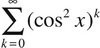, then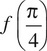is

2. The volume of the solid that results when the area between the graph of y = x2 + 2 and the graph of y = 10 - x2 from x = 0 to x = 2 is rotated around the x-axis is

3.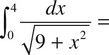4. The rate that an object cools is directly proportional to the difference between its temperature (in Kelvins) at that time and the surrounding temperature (in Kelvins). If an object is initially at 35 K, and the surrounding temperature remains constant at 10 K, it takes 5 minutes for the object to cool to 25 K. How long will it take for the object to cool to 20 K?

5.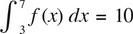and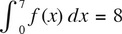find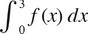6. Two particles leave the origin at the same time and move along the y-axis with their respective positions determined by the functions y1 = cos 2t and y2 = 4sin t for 0 < t < 6. For how many values of t do the particles have the same acceleration?

7. The minimum value of the function y = x3 - 7x + 11, x ≥ 0 is approximately

8. Use Euler’s Method with h = 0.2 to estimate y(1), if y′ = y and y(0) = 1.